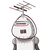# the cost of polishing the top of a circular table of radius 2.1 metre at the rate of 10 rupees per square metre is how much​

the cost of polishing the top of a circular table of radius 2.1 metre at the rate of 10 rupees per square metre is how much​

### 1 thought on “the cost of polishing the top of a circular table of radius 2.1 metre at the rate of 10 rupees per square metre is how much​”

1.Step-by-step explanation:

Area of circle= πr²

= 22/7×21/10×21/10

= 13.86m²

Cost of polishing= ₹10/m²

total cost= ₹10×13.86

= ₹138.6 Ans